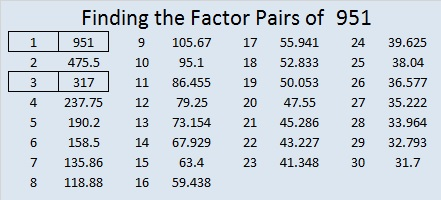# 951 is the 20th Centered Pentagonal Number

Since 951 is the 20th centered pentagonal number, I decided to make the following graphic with 20 concentric pentagons. I’ve outlined the pentagons in the center to make them clearer. The graphic also shows that 951 is one more than five times the 19th triangular number.951 is also the hypotenuse of a Pythagorean triple:
225-924-951 which is 3 times (75-308-317)

As numbers get bigger, palindromes in base 2 get rarer, but 951 is one of them:
1110110111 in BASE 2 because 1(2⁹) + 1(2⁸) + 1(2⁷) + 0(2⁶) + 1(2⁵) + 1(2⁴) + 0(2³) + 1(2²) + 1(2¹) +1(2⁰) = 951
It is also 1D1 in BASE 25 (D is 14 base 10) because 1(25²) + 13(25¹) + 1(25⁰) = 951

• 951 is a composite number.
• Prime factorization: 951 = 3 × 317
• The exponents in the prime factorization are 1 and 1. Adding one to each and multiplying we get (1 + 1)(1 + 1) = 2 × 2 = 4. Therefore 951 has exactly 4 factors.
• Factors of 951: 1, 3, 317, 951
• Factor pairs: 951 = 1 × 951 or 3 × 317
• 951 has no square factors that allow its square root to be simplified. √951 ≈ 30.83828789